Example Questions

← Previous 1

Example Question #84 : Data Analysis

Find the range of the following sequence:

1, 3, 4, 6, 11, 23

6

8

5

22

22

Explanation:

To find the range, subtract the smallest number from the largest number. In this case we get 23 - 1 = 22 so the range is 22.

Example Question #85 : Data Analysis

What is the range of the data set below?

103, 132, 241, 251, 623, 174, 132, 170, 843, 375, 120, 641, 384, 222, 833

833

103

740

473

545

740

Explanation:

The range of a set of data is found by subtracting the smallest item from the largest.  In this case, 843 - 103 = 740.

Example Question #86 : Data Analysis

Find the range of the data set:

25, 83 , 51, 13, 37, 21, 52, 58

42.5

44

83

70

13

70

Explanation:

44 is the median of the data.  42.5 is the arithmetic mean.  13 is the minimum value.  83 is the maximum value.

To find the range, subtract the minimum value from the maximum value:

83 - 13 = 70

Example Question #87 : Data Analysis

F, G, H are the only three numbers in a sequence in which each number is twice the number before it. The three number have an arithmetic mean of 21. What is the value of H?

63

22

24

30

36

36

Explanation:

We know that H = 2G = 4F, and G = 2F and that (F + G + H)/3=21. If we substitute each term with its F equivalent we get:

(F + 2F + 4F)/3 = 21

7F/3 = 21

7F = 63

F = 9

If we substitute F = 9 into H = 4F, we get H = 36.

Example Question #88 : Data Analysis

A set of numbers consists of eleven consecutive even integers. If the median of the set is 62, what is the range of the set?

10
62
52
40
20
Explanation:

We don't know what the numbers are yet, so let's just call them a1, a2, a3, a4....a11. Let's assume a1 is the smallest, and a11 is the greatest.

If we were going to find the median of our set, we would need to line them all up, from least to greatest.

a1, a2, a3, a4, a5, a6, a7, a8, a9, a10, a11

The median is the middle number, which in this case about be a6, because there are five numbers before it and five numbers after it.

We know that the median is 62, so that means that there must be five numbers before 62 and five numbers after. Because the numbers are all consecutive even integers, the set must look like this:

52, 54, 56, 58, 60, 62, 64, 66, 68, 70, 72

To find the range of this set, we must find the difference between the largest and smallest numbers. The largest is 72, and the smallest is 52, so the range is 72 - 52 = 20.

Example Question #1 : Range

The median of a set of eleven consecutive odd integers is 39. What is the range of the set?

29

30

10

20

39

20

Explanation:

Let's represent the eleven integers as A, B, C, D, E, F, G, H, I, J, and K. Let's assume that if we lined them up from least to greatest, the set would be as follows:

A, B, C, D, E, F, G, H, I, J, K

The median in this set is the middle number. In this case, the middle number will always be F, because there are five numbers before it and five numbers after it.

We are told that the median is 39. Thus F = 39, and our set looks like this:

A, B, C, D, E, 39, G, H, I, J, K

Since we know that all the numbers are consecutive odd integers, the set must consist of the five odd numbers before 39 and the five odd numbers after 39. In other words, the set is this:

29, 31, 33, 35, 37, 39, 41, 43, 45, 47, 49

The question asks for the range of the set, which is the difference between the largest and smallest numbers. In this case, the range is 49 – 29 = 20.

Example Question #2 : Range

Find the range.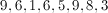Explanation:

First, arrange the numbers from highest to lowest, then subtract the highest number from the lowest number.

Example Question #3 : Range

You have the following data set: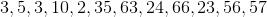What is the range of the data set?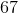Explanation:

The range can be obtained by subtracting the lowest number in the set from the highest number in the set.

The lowest number in the set is 2 and the highest number is 66.

Therefore,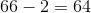is the range of the data set.

Example Question #4 : Range

The following numbers are selected from a set of data: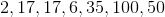Which of the following could NOT be the range of the data set?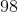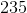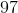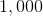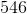Explanation:

The range of the data is the difference between the largest number and the smallest number. The range of the given data is; therefore, the range of the entire data set must be equal to or greater than. So, it could not be.

Example Question #5 : Range

What is the range of the following data set?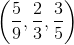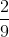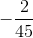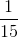Explanation:

Determine the smallest and largest fractions by rewriting all the fractions to a similar denominator.

Multiply all three denominators together to get the least common denominator, and multiply the numerators by what was multiplied by the denominator to obtain the new numerators.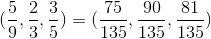It can then be seen that the smallest fraction is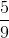and the largest fraction is.

Write the range formula and solve.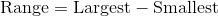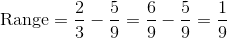← Previous 1